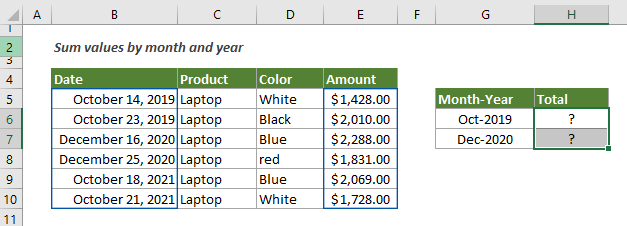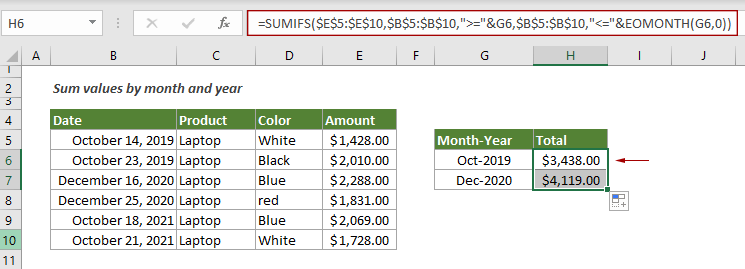## Sum values by month (with or without year) in Excel

This tutorial demonstrates two formulas in detail to help summing values by certain month and year or summing values by certain month ignore year in Excel.

How to sum values by month and year?
How to sum values by month ignore year?

#### How to sum values by month and year?

As shown in the screenshot below, to sum the total amount based on October from the same year (2019), you can use a formula with the SUMIFS and EOMONTH functions.Generic Formulas

=SUMIFS(value_range,date_range,">="&date,date_range,"<="&EOMONTH(date,0))

Arguments

Value_range: The range of values you want to sum;
Date_range: The date range where you want to evaluate the month and year;
Date: Represents the first day of a month you want to sum based on. It can be a cell reference or an exact date enclosed in double quotation marks.

How to use this formula?

In this case, \$D\$3:\$D\$11 is the range1, China is the criteria1 that locates in \$G3, \$E\$3:\$E\$11 is the range2, and H\$2 is the cell reference containing the criteria2.

1. Select a blank cell to output the result. In this case, I select H6.

2. Copy or enter the below formula into the selected cell and press the Enter key. Select the result cell, drag the AutoFill Handle down to get the sum by other month and year.

=SUMIFS(\$E\$5:\$E\$10,\$B\$5:\$B\$10,">="&G6,\$B\$5:\$B\$10,"<="&EOMONTH(G6,0))Note: In range G6:G7, I entered actual dates in “m/d/yyyy” format into the cells and then manually formatted the dates as “mmm-yyyy” to only display the month and year. If you manually enter the month and year as text into the cell, you will not get the correct result.

Explanation of the formula

=SUMIFS(\$E\$5:\$E\$10,\$B\$5:\$B\$10,">="&G6,\$B\$5:\$B\$10,"<="&EOMONTH(G6,0))

\$E\$5:\$E\$10,\$B\$5:\$B\$10,">="&G6: Here match dates in the date range E5:E10 greater than or equal to the first day of a month in G6.
\$B\$5:\$B\$10,"<="&EOMONTH(G6,0): The EOMONTH function returns the serial number for the last day of the month provided by the date in G6. As above, here match dates in the date range E5:E10 less than or equal to the last day of the month.
Then the SUMIFS function sums the total amount by month with the above two criteria.

#### How to sum values by month ignore year?

With the above formula, you can only sum values by month from the same year. To sum values by month from different years, you can apply a formula based on the SUMPROFUCT and MONTH functions.

Generic formula

=SUMPRODUCT((MONTH(date_range)=month)*value_range)

Arguments

Date_range: The date range where you want to evaluate the month;
Month: It is a number representing the month that you will sum values based on;
Value_range: The range of values you want to sum;

How to use this formula?

1. Select a blank cell. Here I select H6.

2. Copy or enter the below formula into it and press the Enter key to get the result.

=SUMPRODUCT((MONTH(\$B\$5:\$B\$10)=10)*\$E\$5:\$E\$10)

Note: In the formula, the number 10 represents the month October. The numbers 1 to 12 separately represent January to December, and you can change it according to your needs.

Explanation of the formula

=SUMPRODUCT((MONTH(\$B\$5:\$B\$10)=10)*\$E\$5:\$E\$10)

MONTH(\$B\$5:\$B\$10)=10: The MONTH function extracts the month number from each date cell in the range B5:B10: {10;10;12;12;10;10}, and compare each number in the array with the number 10: {10;10;12;12;10;10}=10, finally it returns a TRUE FALSE array {TRUE;TRUE;FALSE;FALSE;TRUE;TRUE}.
{TRUE;TRUE;FALSE;FALSE;TRUE;TRUE}*\$E\$5:\$E\$10: This array is multiplied by each amount associated with the month October, and you will get a new array like this {1428;2010;0;0;2069;1728};
SUMPRODUCT{1428;2010;0;0;2069;1728}: The SUMPRODUCT function finally adds up all numbers in the array and returns the result as 7235.

#### Related functions

Excel SUMIFS function
The Excel SUMIFS function helps to sum cell values based on multiple criteria

Excel SUMPRODUCT function
The Excel SUMPRODUCT function can be used to multiply two or more columns or arrays together, and then get the sum of products.

Excel EOMONTH function
The Excel EOMONTH function gets the date that is a specific number of months before or after a given start date

Excel MONTH function
The Excel MONTH function extracts the month number from a date format in Excel

#### Related formulas

Sum values by the week of a given date
This tutorial talks about how to sum values by the week in which a given date falls

Sum values by weeknum or weekday
This tutorial demonstrates formulas step-by-step to help you sum values in a certain range based on the specified week number or weekday in Excel.

Sum every n rows or columns in a range
This tutorial explains two formulas based on the SUM and OFFSET functions to sum every n rows or columns in a specified range in Excel.

Sum every nth row or column in a range
This tutorial is going to show you how to create a formula based on the SUMPRODUCT, MOD and COLUMN functions to sum every nth row or column in a range in Excel.

### The Best Office Productivity Tools

#### Kutools for Excel - Helps You To Stand Out From Crowd

 Popular Features: Find, Highlight or Identify Duplicates  |  Delete Blank Rows  |  Combine Columns or Cells without Losing Data  |  Round without Formula ... Super VLookup: Multiple Criteria  |  Multiple Value  |  Across Multi-Sheets  |  Fuzzy Lookup... Adv. Drop-down List: Easy Drop Down List  |  Dependent Drop Down List  |  Multi-select Drop Down List... Column Manager: Add a Specific Number of Columns  |  Move Columns  |  Toggle Visibility Status of Hidden Columns  |  Compare Columns to Select Same & Different Cells ... Featured Features: Grid Focus  |  Design View  |  Big Formula Bar  |  Workbook & Sheet Manager | Resource Library (Auto Text)  |  Date Picker  |  Combine Worksheets  |  Encrypt/Decrypt Cells  |  Send Emails by List  |  Super Filter  |  Special Filter (filter bold/italic/strikethrough...) ... Top 15 Toolsets:  12 Text Tools (Add Text, Remove Characters ...)  |  50+ Chart Types (Gantt Chart ...)  |  40+ Practical Formulas (Calculate age based on birthday ...)  |  19 Insertion Tools (Insert QR Code, Insert Picture from Path ...)  |  12 Conversion Tools (Numbers to Words, Currency Conversion ...)  |  7 Merge & Split Tools (Advanced Combine Rows, Split Excel Cells ...)  |  ... and more

Kutools for Excel Boasts Over 300 Features, Ensuring That What You Need is Just A Click Away...#### Office Tab - Enable Tabbed Reading and Editing in Microsoft Office (include Excel)

• One second to switch between dozens of open documents!
• Reduce hundreds of mouse clicks for you every day, say goodbye to mouse hand.
• Increases your productivity by 50% when viewing and editing multiple documents.
• Brings Efficient Tabs to Office (include Excel), Just Like Chrome, Edge and Firefox.